# Geometric Waves

Simple geometric waves are often used in sound synthesis since they have a rich complement of harmonics. These harmonics can be filtered to produce a variety of sounds.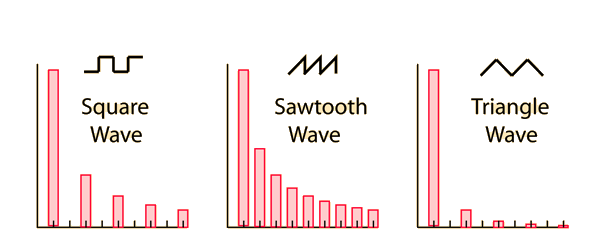Click on any of the waves for further details.
 Fourier analysis and synthesis
Index

Sound reproduction concepts

 HyperPhysics***** Sound R Nave
Go Back

# Square Wave

 The square wave contains only odd harmonics with the amplitudes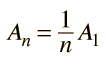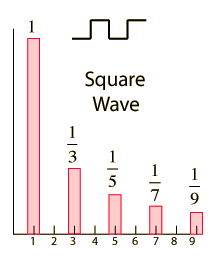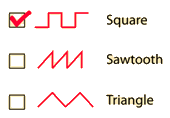Discussion of geometric waves
Index

Sound reproduction concepts

 HyperPhysics***** Sound R Nave
Go Back

# Sawtooth Wave

 The sawtooth wave is useful for synthesis since it contains all harmonics in the geometric ratio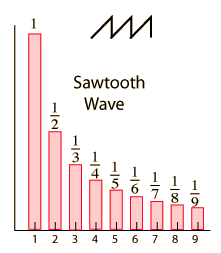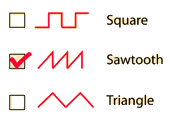Discussion of geometric waves
Index

Sound reproduction concepts

 HyperPhysics***** Sound R Nave
Go Back

<

# Triangle Wave

 The triangle wave contains only odd harmonics with the amplitudes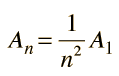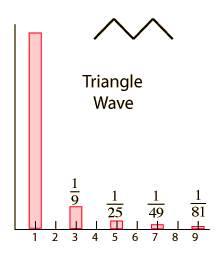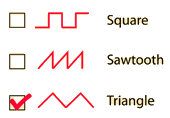Discussion of geometric waves
Index

Sound reproduction concepts

 HyperPhysics***** Sound R Nave
Go Back